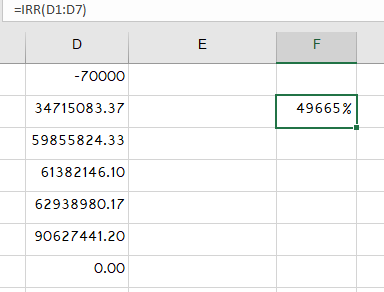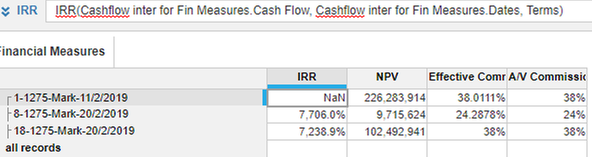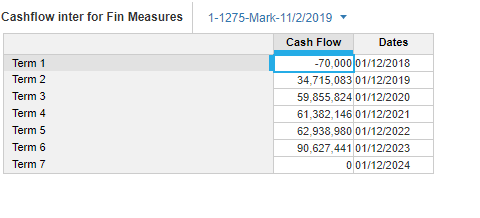# Used IRR formula in a Model but it is returning NaN as result whereas when Same numbers are given asUsed IRR formula in a Model but it is returning NaN as result whereas when Same numbers are given as a input to IRR formula in Excel it is returning a numbeTagged: